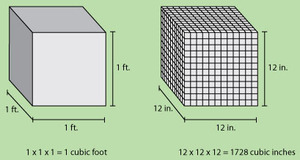# Unit Conversions

Alignments to Content Standards: 6.RP.A.3.d

There are many resources available on the computer to help converting between different units. This task examines a few important aspects of some of these conversions.

1. According to an online calculator, there are 1728 cubic inches in a cubic foot.
1. Explain how to check the calculator's answer. Is it correct?
2. How many cubic inches are there in 5 cubic feet? What about in 200 cubic feet? Explain how to find these answers without the calculator using the number of cubic inches in one cubic foot.
2. A given website converts kilograms to pounds allowing the user to select how many decimals to provide in the conversion. With no decimals it says ''1 kilogram = 2 pounds.'' For one decimal, it says ''1 kilogram = 2.2 pounds.''
1. According to these two conversion rates, how many pounds are in 5 kilograms?
2. What would be a better way of stating these conversion rates?

## IM Commentary

The goal of this task is to study conversion between some volume and weight units. There are many important aspects to these conversions, including:

• Gaining an intuition for the meaning of different units of measure. How big, in every day terms, is a cubic centimeter or cubic millimeter? This intuition can then be used to
• Assess the reasonableness of an answer: is it reasonable that there are about 30 centimeters in a foot?
• Understanding the relationship between multiplication, linear measurements, area, and volume.

The focus of this task is on the last of these but the teacher may wish to take time to let the students experiment with an online conversion program if computer resources are available. For part (a) of this task http://www.asknumbers.com/VolumeConversion.aspx is one online resource while http://www.asknumbers.com/WeightConversion.aspx was used for part (b). Students will perhaps enjoy learning about some of the different measures which they have probably not heard of before, such as 'hogshead' and 'jigger' for volume or 'parsec' and 'dyne' for mass. The picture for part (a) of the solution is taken from a document created by the Dana Center: http://www.utdanacenter.org/k12mathbenchmarks/elementary/measurement.php.  The actual conversion from pounds to kilograms is indeed rather subtle, as one of the units takes into account the strength of gravity, and so varies based on your location on earth.  Nonetheless, the most standardly quoted conversion factor is that 1 kilogram is approximately 2.20462 pounds.

The first part of the question builds on student knowledge of 6.G.2, relating volume to multiplication of length, width, and height. After finding out how many cubic inches are in a cubic foot, students then apply knowledge of ratios to make additional conversions from cubic inches to cubic feet. The second question gives students an opportunity to think about precision in calculation. They may also wonder what exactly a centimeter or an inch is and might be surprised to find out how technical the definitions of these lengths are.

## Solution

1. In order to check the online calculator's answer of 1728 cubic inches per cubic foot, we start with a picture:The picture shows a cubic foot divided into cubic inches. There are 12 (vertical) layers, each one inch high. These layers are made up of 12 rows of cubes, each containing 12 cubes. So each layer has 12 $\times$ 12 unit cubes and, since there are 12 layers, there are 12 $\times$ (12 $\times$ 12) total cubic inches in the cubic foot.

2. To find the number of cubic inches in 5 cubic feet and 200 cubic feet (or any number of cubic feet) we can make a ratio table:
Cubic Feet Cubic Inches
1 1728
5 8640
200 345,600

To obtain the second row, for example, we have \begin{align} 5 \text{ cubic feet} \times 1728 \frac{\text{cubic inches}}{\text{cubic foot}} &= 5 \times 1728 \text{ cubic inches}\\ &=  8640 \text{ cubic inches}.\end{align}

1. If there are 2 pounds in a kilogram then in 5 kilograms we will have 5 $\times$ 2 = 10 pounds. If there are 2.2 pounds in a kilogram then in 5 kilograms there are 11 pounds: \begin{align} 5 \text{ kilograms} \times 2.2 \frac{\text{pounds}}{\text{kilogram}} &= 5 \times 2.2 \text{ pounds}\\ &=  11 \text{ pounds}.\end{align} These values do not agree.
2. In each case, the conversions are only approximate. The exact number of pounds in a kilogram is very complicated and so in practice we deal with an approximation which is accurate enough to suit our needs. To make this clearer, we can use an approximately symbol, writing 1 kilogram $\approx$ 2 pounds, or 1 kilogram $\approx$ 2.2 pounds.